# RD Sharma Solutions for Class 11 Chapter 12 - Mathematical Induction Exercise 12.2

This exercise deals with problems based on the second principle of mathematical induction. Students who aim to score high marks are advised to solve the exercise wise problems using the solutions pdf as a reference guide. Solving the problems improves analytical thinking and problem-solving abilities among students. Hence, by practising the solutions on a regular basis, students can top their board exams. RD Sharma Class 11 Solutions Maths is available in the pdf format, students can easily download the pdf from the links given below and can start practising offline.

## Download the Pdf of RD Sharma Solutions for Class 11 Maths Exercise 12.2 Chapter 12 – Mathematical Induction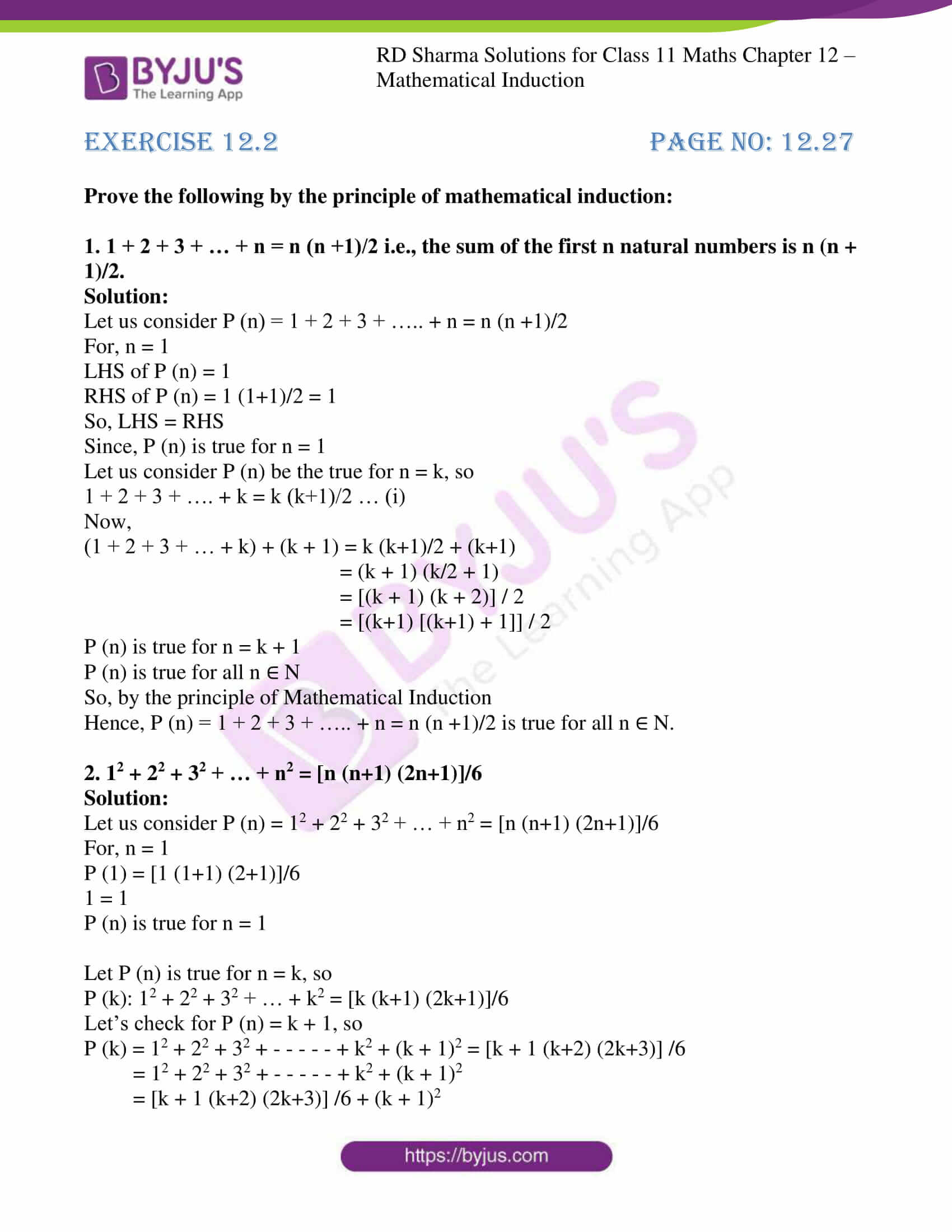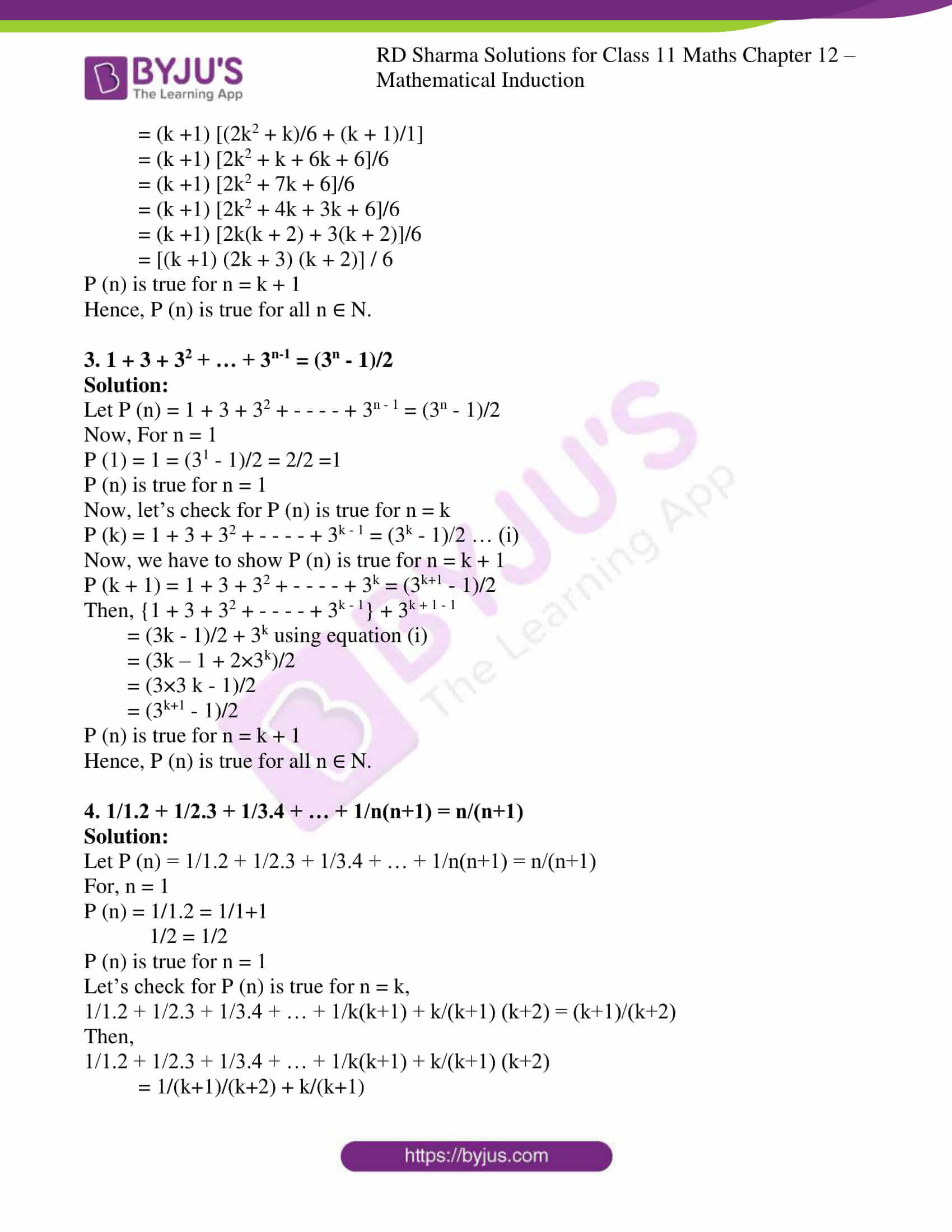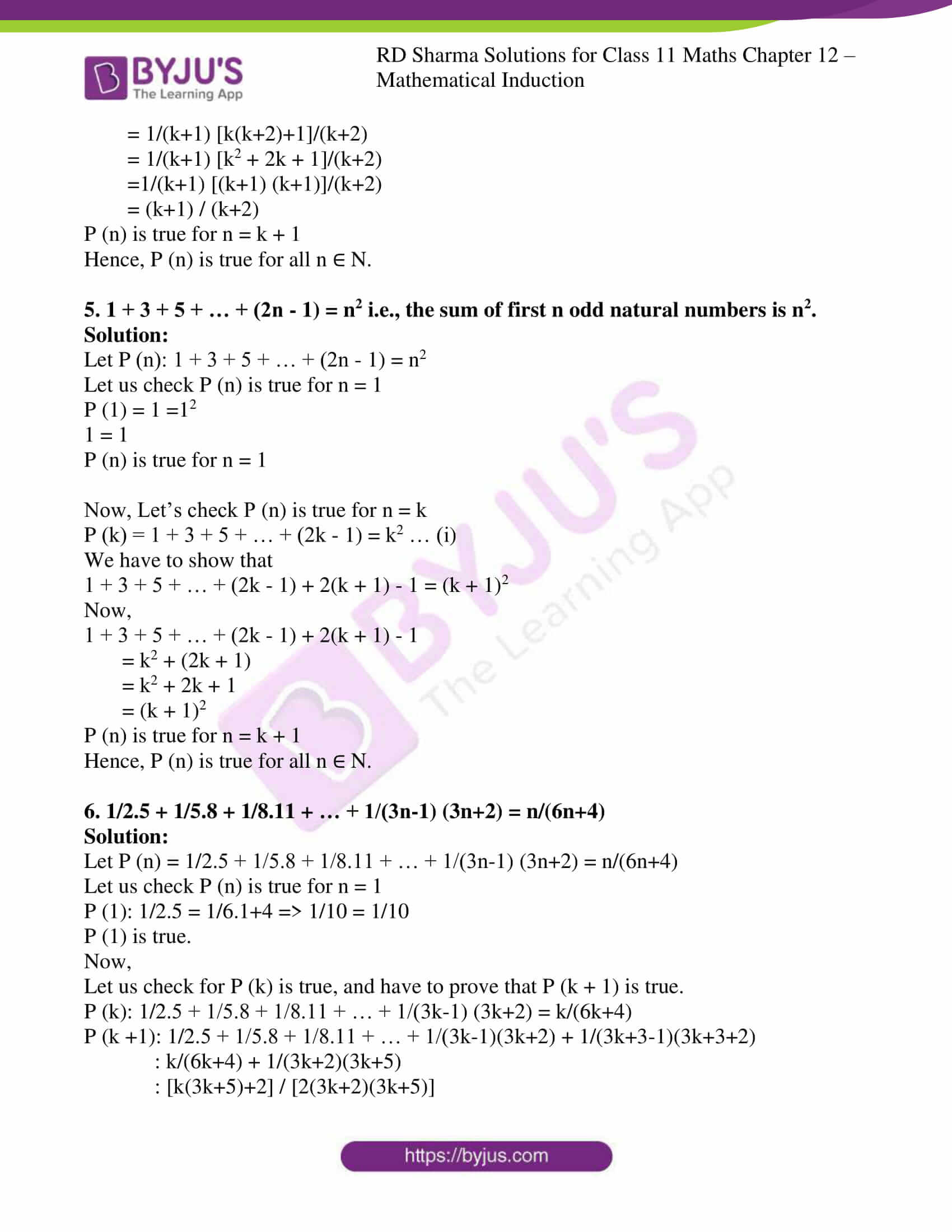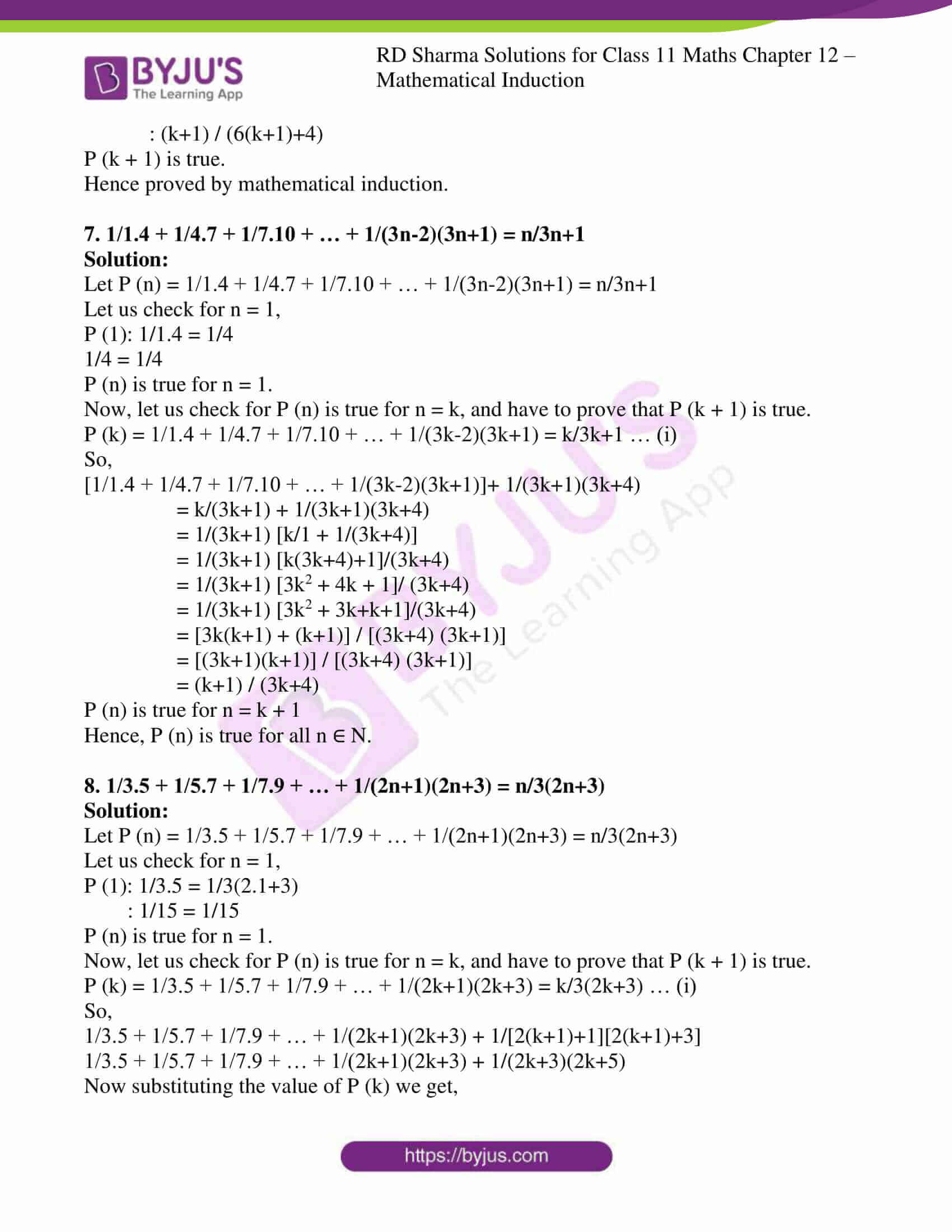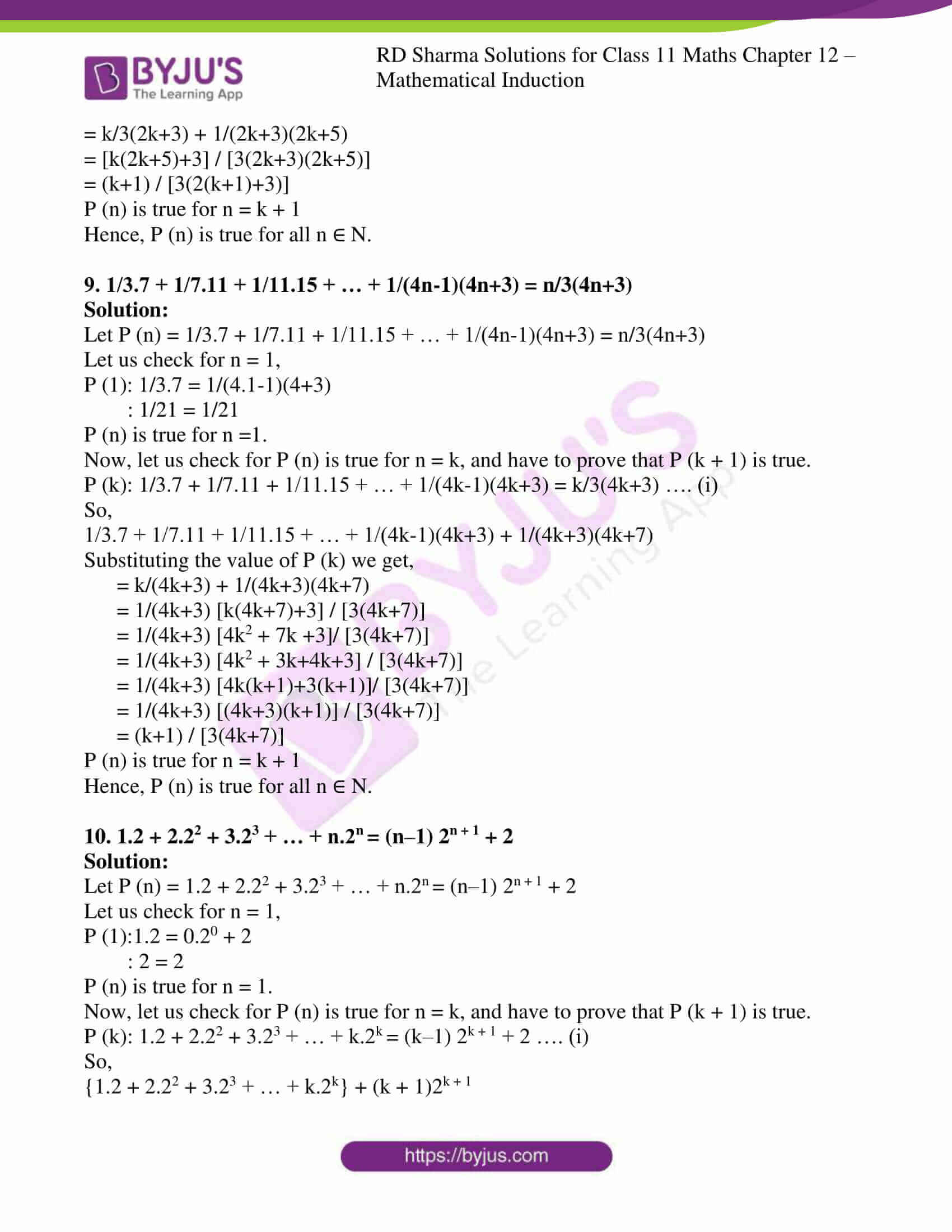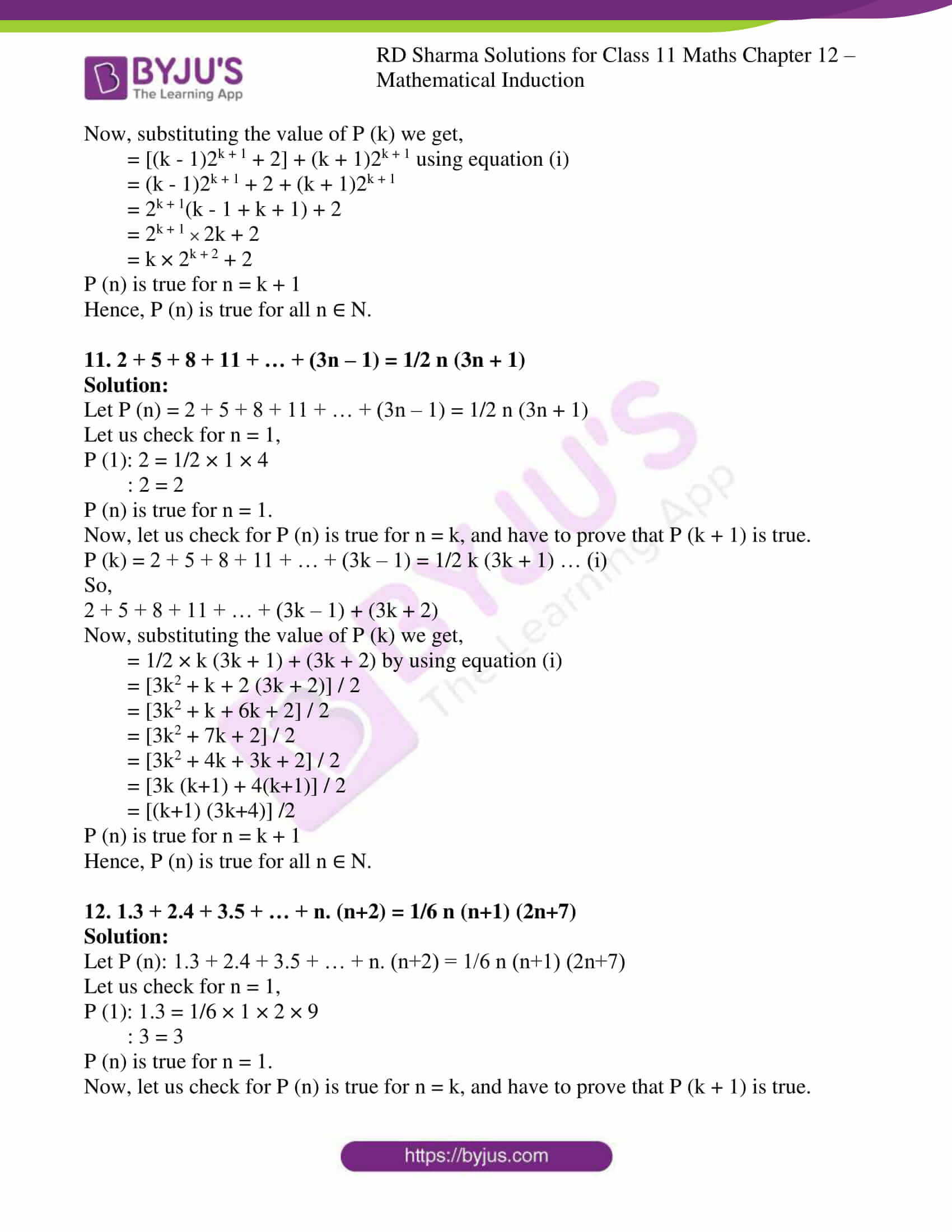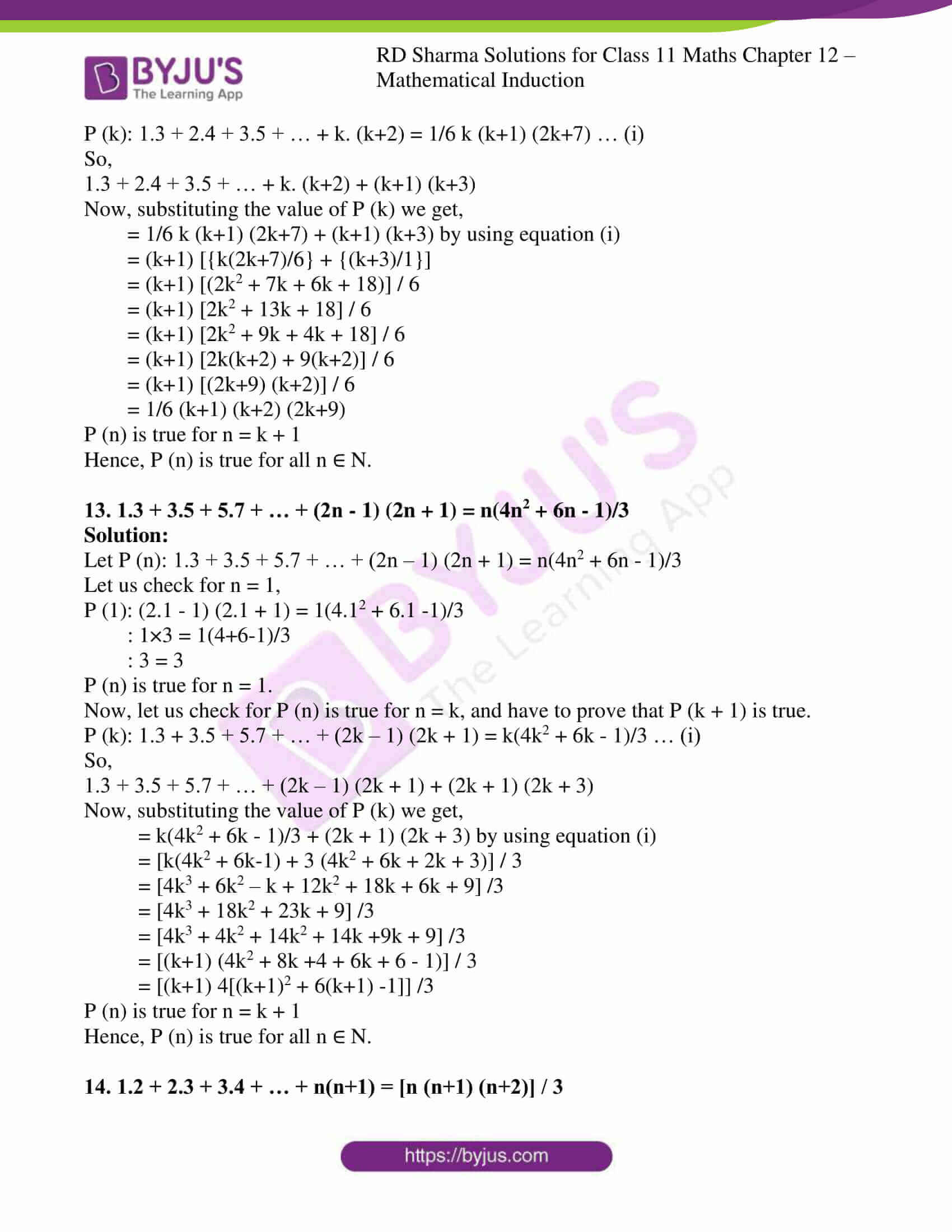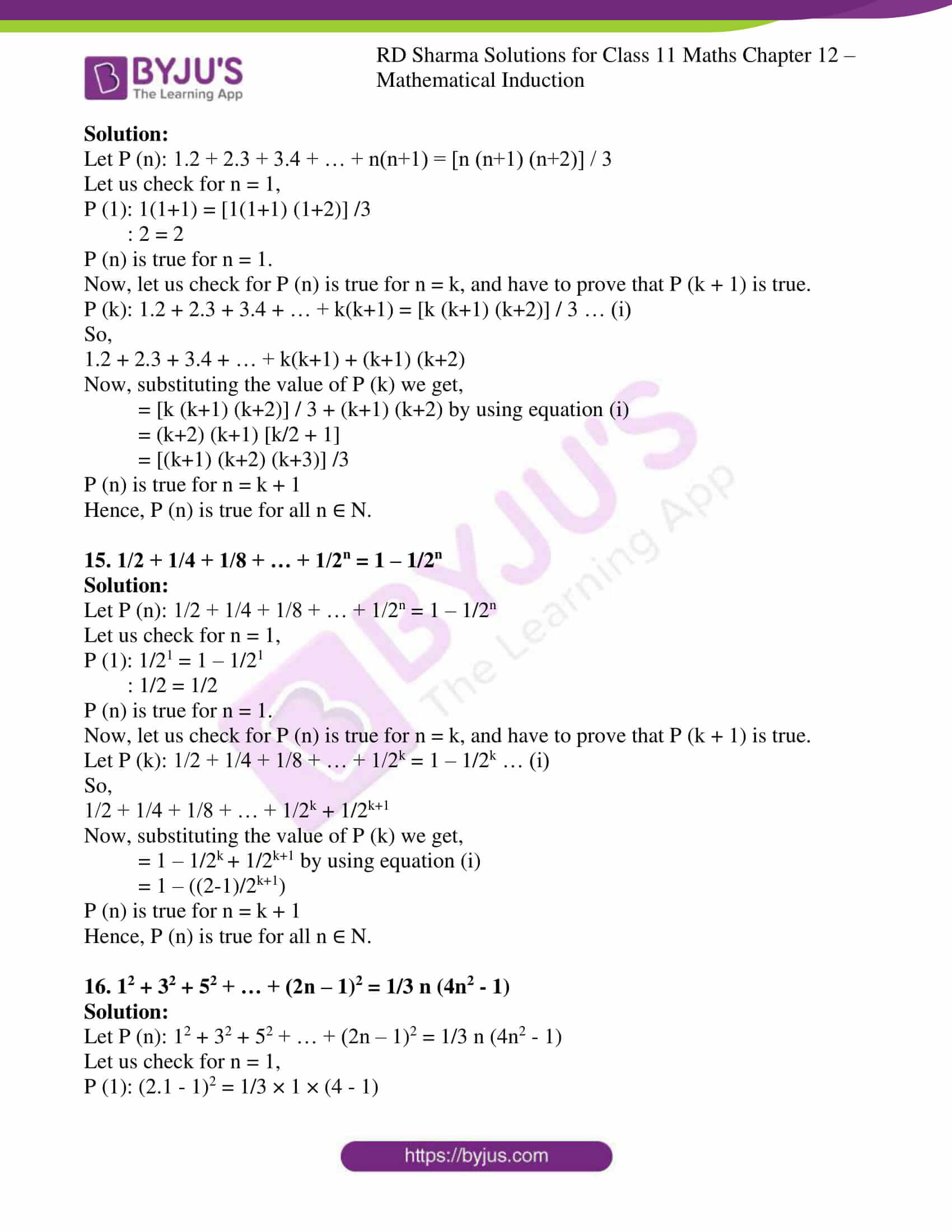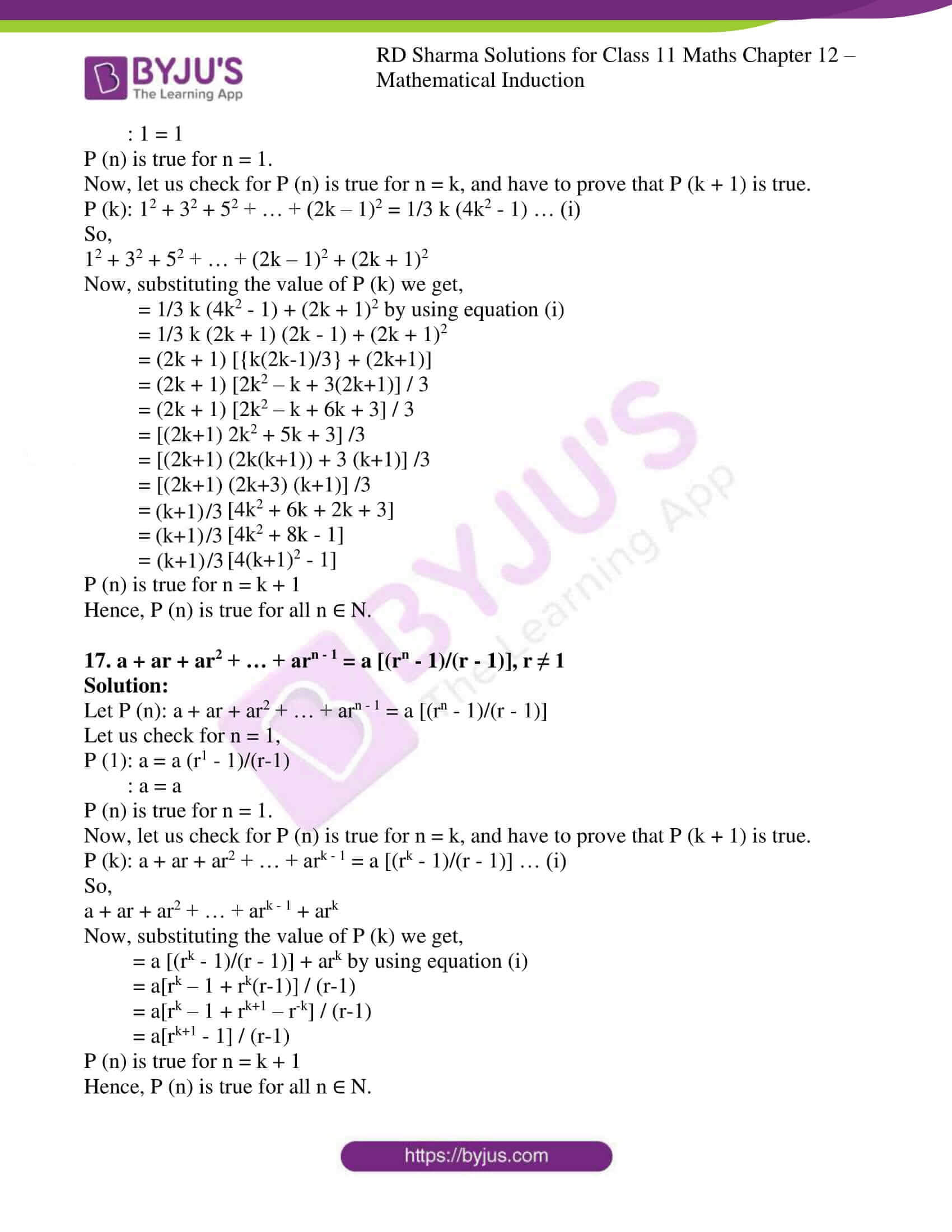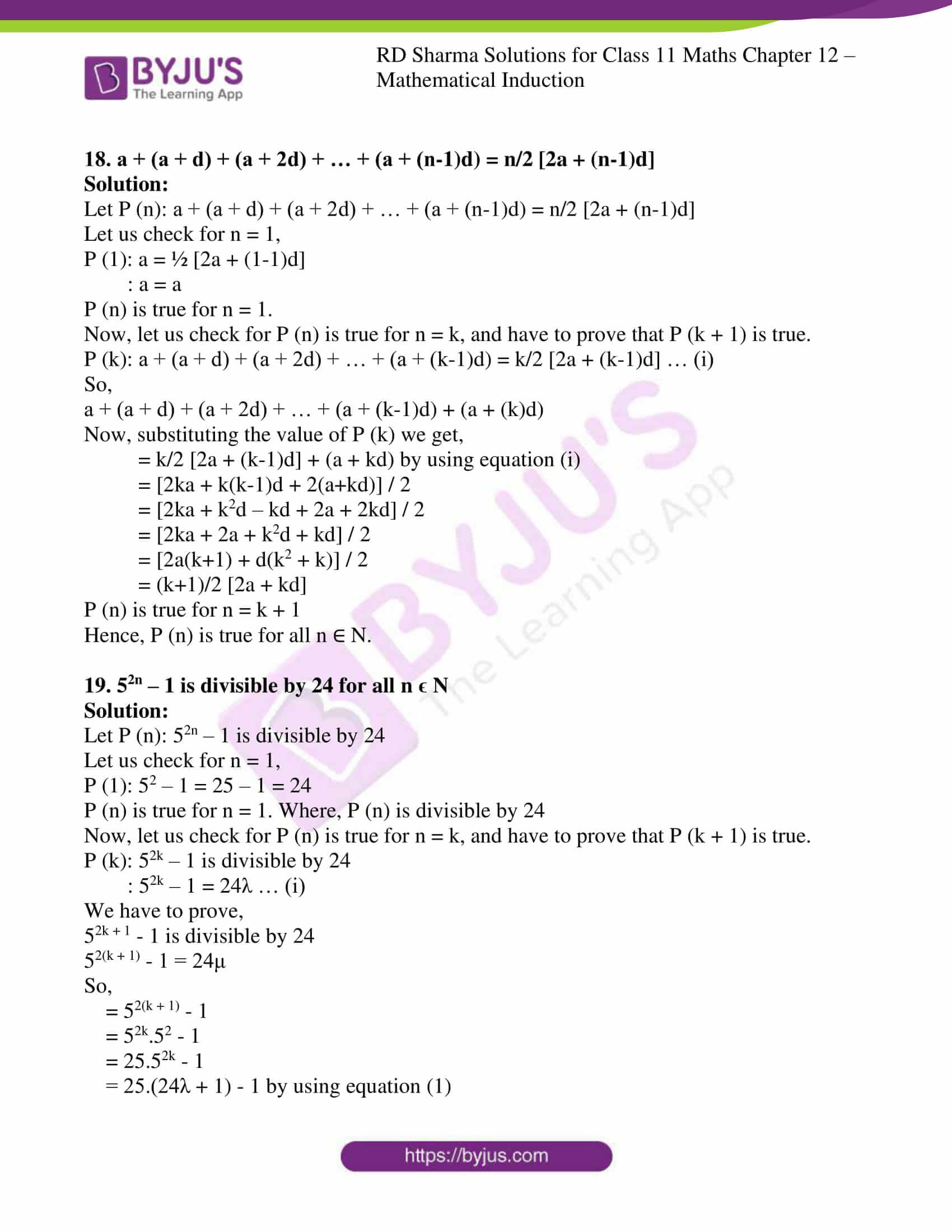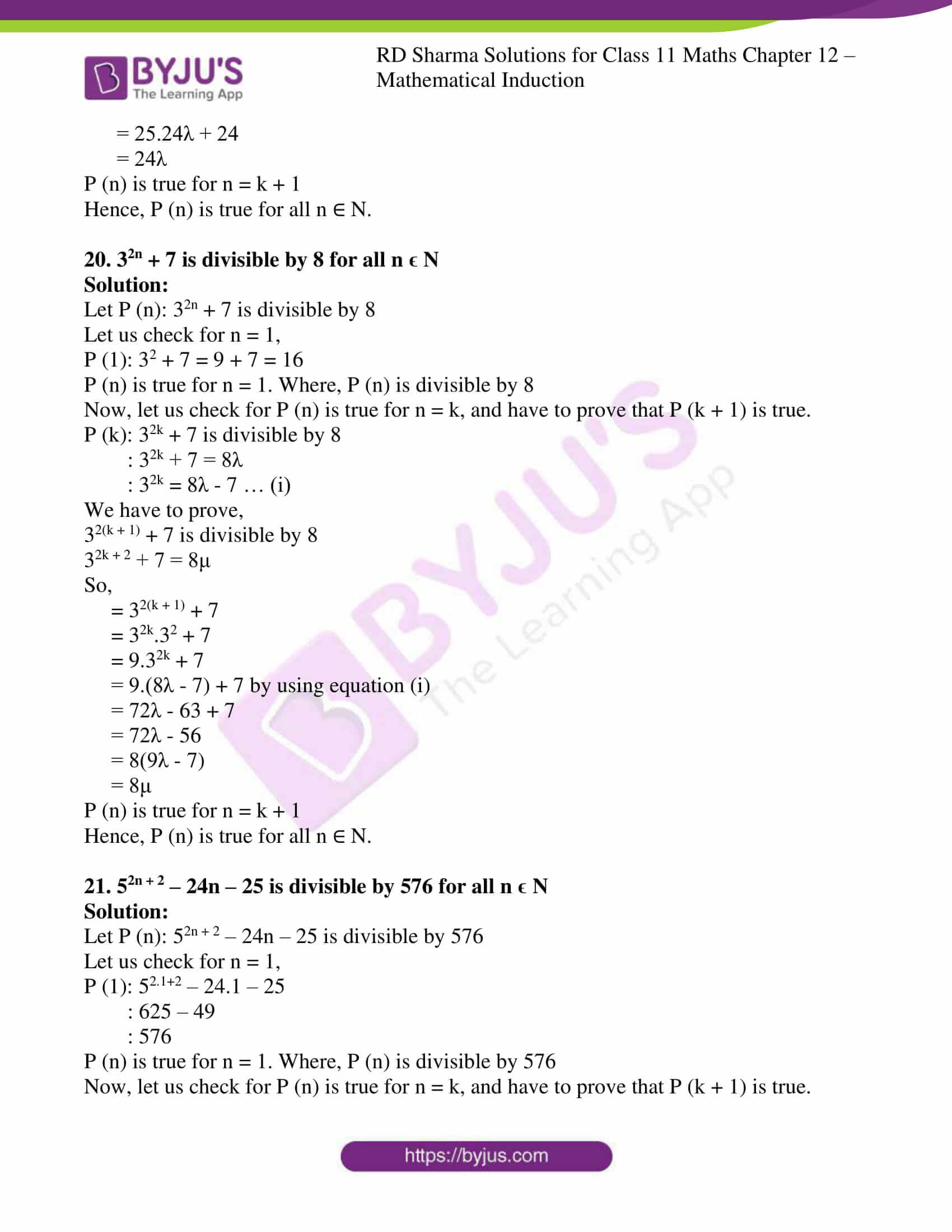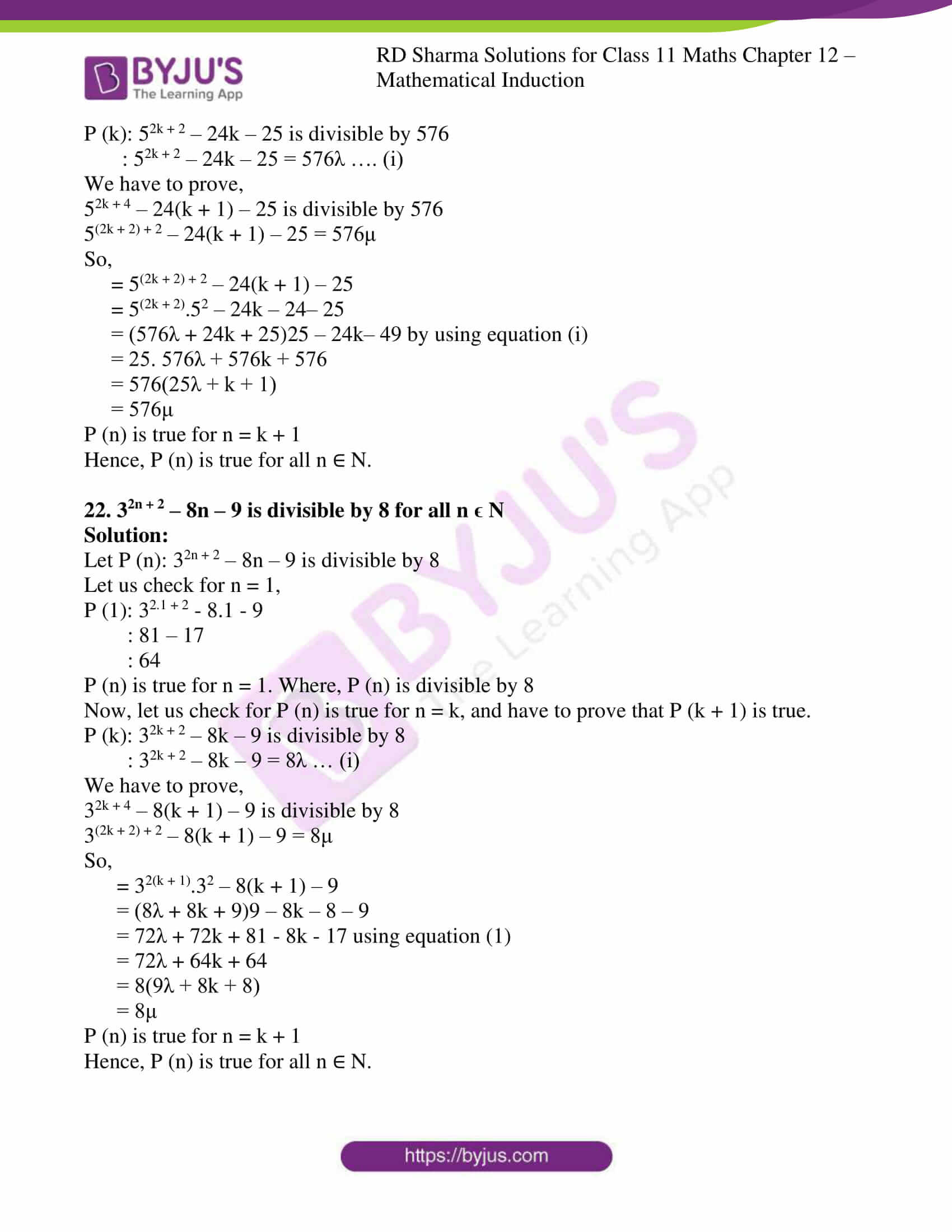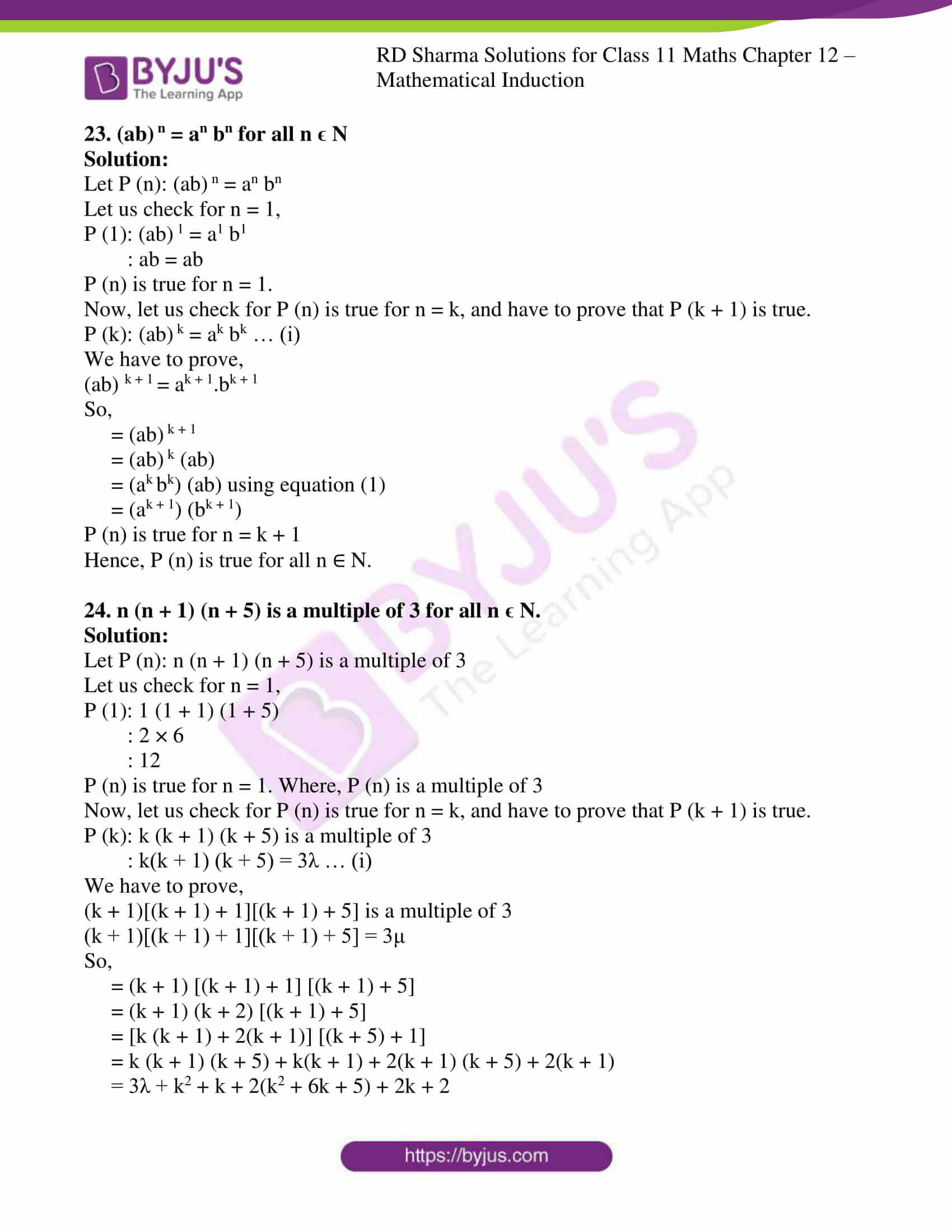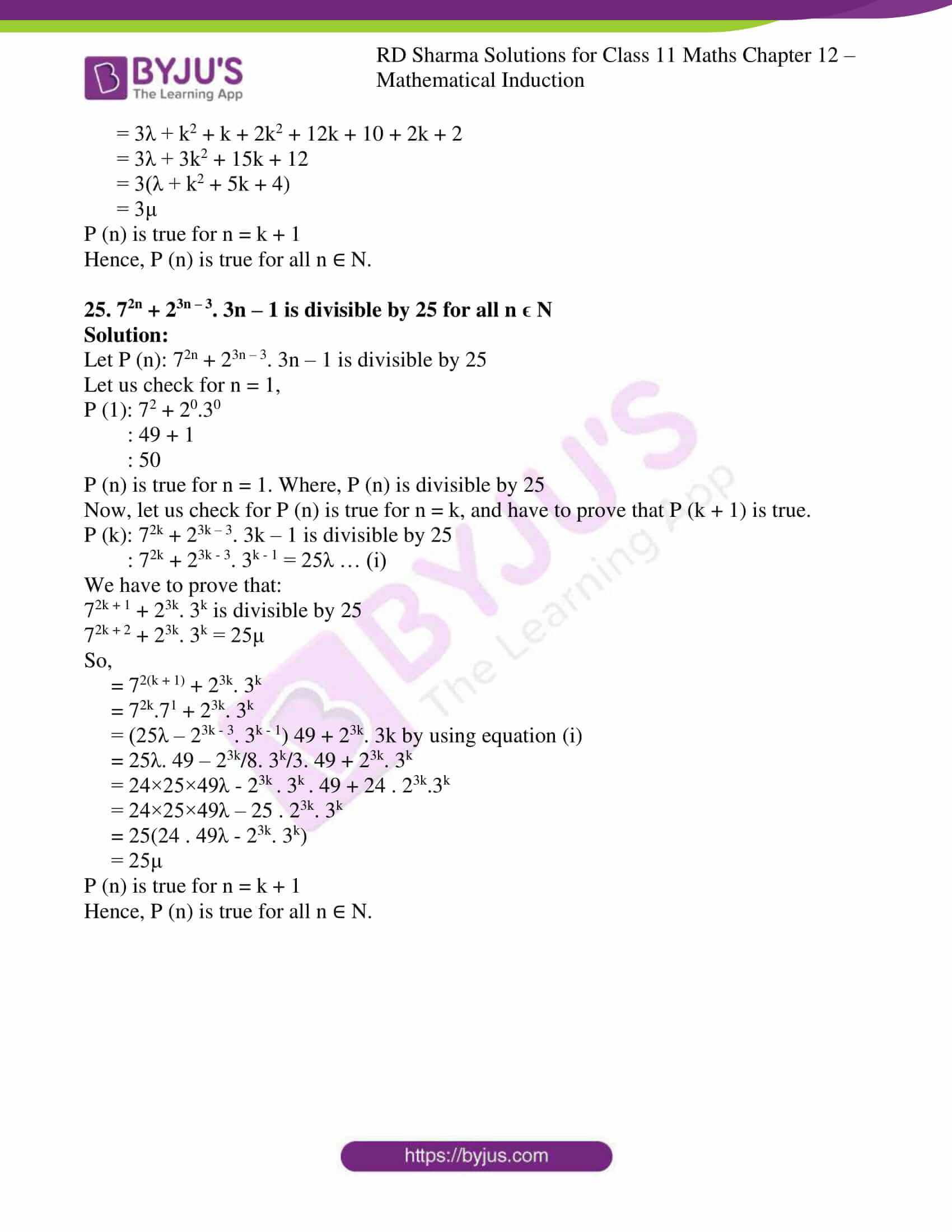### Also, access another exercise of RD Sharma Solutions for Class 11 Maths Chapter 12 – Mathematical Induction

Exercise 12.1 Solutions

### Access answers to RD Sharma Solutions for Class 11 Maths Exercise 12.2 Chapter 12 – Mathematical Induction

Prove the following by the principle of mathematical induction:

1. 1 + 2 + 3 + … + n = n (n +1)/2 i.e., the sum of the first n natural numbers is n (n + 1)/2.

Solution:

Let us consider P (n) = 1 + 2 + 3 + ….. + n = n (n +1)/2

For, n = 1

LHS of P (n) = 1

RHS of P (n) = 1 (1+1)/2 = 1

So, LHS = RHS

Since, P (n) is true for n = 1

Let us consider P (n) be the true for n = k, so

1 + 2 + 3 + …. + k = k (k+1)/2 … (i)

Now,

(1 + 2 + 3 + … + k) + (k + 1) = k (k+1)/2 + (k+1)

= (k + 1) (k/2 + 1)

= [(k + 1) (k + 2)] / 2

= [(k+1) [(k+1) + 1]] / 2

P (n) is true for n = k + 1

P (n) is true for all n ∈ N

So, by the principle of Mathematical Induction

Hence, P (n) = 1 + 2 + 3 + ….. + n = n (n +1)/2 is true for all n ∈ N.

2. 12 + 22 + 32 + … + n2 = [n (n+1) (2n+1)]/6

Solution:

Let us consider P (n) = 12 + 22 + 32 + … + n2 = [n (n+1) (2n+1)]/6

For, n = 1

P (1) = [1 (1+1) (2+1)]/6

1 = 1

P (n) is true for n = 1

Let P (n) is true for n = k, so

P (k): 12 + 22 + 32 + … + k2 = [k (k+1) (2k+1)]/6

Let’s check for P (n) = k + 1, so

P (k) = 12 + 22 + 32 + – – – – – + k2 + (k + 1)2 = [k + 1 (k+2) (2k+3)] /6

= 12 + 22 + 32 + – – – – – + k2 + (k + 1)2

= [k + 1 (k+2) (2k+3)] /6 + (k + 1)2

= (k +1) [(2k2 + k)/6 + (k + 1)/1]

= (k +1) [2k2 + k + 6k + 6]/6

= (k +1) [2k2 + 7k + 6]/6

= (k +1) [2k2 + 4k + 3k + 6]/6

= (k +1) [2k(k + 2) + 3(k + 2)]/6

= [(k +1) (2k + 3) (k + 2)] / 6

P (n) is true for n = k + 1

Hence, P (n) is true for all n ∈ N.

3. 1 + 3 + 32 + … + 3n-1 = (3n – 1)/2

Solution:

Let P (n) = 1 + 3 + 32 + – – – – + 3n – 1 = (3n – 1)/2

Now, For n = 1

P (1) = 1 = (31 – 1)/2 = 2/2 =1

P (n) is true for n = 1

Now, let’s check for P (n) is true for n = k

P (k) = 1 + 3 + 32 + – – – – + 3k – 1 = (3k – 1)/2 … (i)

Now, we have to show P (n) is true for n = k + 1

P (k + 1) = 1 + 3 + 32 + – – – – + 3k = (3k+1 – 1)/2

Then, {1 + 3 + 32 + – – – – + 3k – 1} + 3k + 1 – 1

= (3k – 1)/2 + 3k using equation (i)

= (3k – 1 + 2×3k)/2

= (3×3 k – 1)/2

= (3k+1 – 1)/2

P (n) is true for n = k + 1

Hence, P (n) is true for all n ∈ N.

4. 1/1.2 + 1/2.3 + 1/3.4 + … + 1/n(n+1) = n/(n+1)

Solution:

Let P (n) = 1/1.2 + 1/2.3 + 1/3.4 + … + 1/n(n+1) = n/(n+1)

For, n = 1

P (n) = 1/1.2 = 1/1+1

1/2 = 1/2

P (n) is true for n = 1

Let’s check for P (n) is true for n = k,

1/1.2 + 1/2.3 + 1/3.4 + … + 1/k(k+1) + k/(k+1) (k+2) = (k+1)/(k+2)

Then,

1/1.2 + 1/2.3 + 1/3.4 + … + 1/k(k+1) + k/(k+1) (k+2)

= 1/(k+1)/(k+2) + k/(k+1)

= 1/(k+1) [k(k+2)+1]/(k+2)

= 1/(k+1) [k2 + 2k + 1]/(k+2)

=1/(k+1) [(k+1) (k+1)]/(k+2)

= (k+1) / (k+2)

P (n) is true for n = k + 1

Hence, P (n) is true for all n ∈ N.

5. 1 + 3 + 5 + … + (2n – 1) = n2 i.e., the sum of first n odd natural numbers is n2.

Solution:

Let P (n): 1 + 3 + 5 + … + (2n – 1) = n2

Let us check P (n) is true for n = 1

P (1) = 1 =12

1 = 1

P (n) is true for n = 1

Now, Let’s check P (n) is true for n = k

P (k) = 1 + 3 + 5 + … + (2k – 1) = k2 … (i)

We have to show that

1 + 3 + 5 + … + (2k – 1) + 2(k + 1) – 1 = (k + 1)2

Now,

1 + 3 + 5 + … + (2k – 1) + 2(k + 1) – 1

= k2 + (2k + 1)

= k2 + 2k + 1

= (k + 1)2

P (n) is true for n = k + 1

Hence, P (n) is true for all n ∈ N.

6. 1/2.5 + 1/5.8 + 1/8.11 + … + 1/(3n-1) (3n+2) = n/(6n+4)

Solution:

Let P (n) = 1/2.5 + 1/5.8 + 1/8.11 + … + 1/(3n-1) (3n+2) = n/(6n+4)

Let us check P (n) is true for n = 1

P (1): 1/2.5 = 1/6.1+4 => 1/10 = 1/10

P (1) is true.

Now,

Let us check for P (k) is true, and have to prove that P (k + 1) is true.

P (k): 1/2.5 + 1/5.8 + 1/8.11 + … + 1/(3k-1) (3k+2) = k/(6k+4)

P (k +1): 1/2.5 + 1/5.8 + 1/8.11 + … + 1/(3k-1)(3k+2) + 1/(3k+3-1)(3k+3+2)

: k/(6k+4) + 1/(3k+2)(3k+5)

: [k(3k+5)+2] / [2(3k+2)(3k+5)]

: (k+1) / (6(k+1)+4)

P (k + 1) is true.

Hence proved by mathematical induction.

7. 1/1.4 + 1/4.7 + 1/7.10 + … + 1/(3n-2)(3n+1) = n/3n+1

Solution:

Let P (n) = 1/1.4 + 1/4.7 + 1/7.10 + … + 1/(3n-2)(3n+1) = n/3n+1

Let us check for n = 1,

P (1): 1/1.4 = 1/4

1/4 = 1/4

P (n) is true for n = 1.

Now, let us check for P (n) is true for n = k, and have to prove that P (k + 1) is true.

P (k) = 1/1.4 + 1/4.7 + 1/7.10 + … + 1/(3k-2)(3k+1) = k/3k+1 … (i)

So,

[1/1.4 + 1/4.7 + 1/7.10 + … + 1/(3k-2)(3k+1)]+ 1/(3k+1)(3k+4)

= k/(3k+1) + 1/(3k+1)(3k+4)

= 1/(3k+1) [k/1 + 1/(3k+4)]

= 1/(3k+1) [k(3k+4)+1]/(3k+4)

= 1/(3k+1) [3k2 + 4k + 1]/ (3k+4)

= 1/(3k+1) [3k2 + 3k+k+1]/(3k+4)

= [3k(k+1) + (k+1)] / [(3k+4) (3k+1)]

= [(3k+1)(k+1)] / [(3k+4) (3k+1)]

= (k+1) / (3k+4)

P (n) is true for n = k + 1

Hence, P (n) is true for all n ∈ N.

8. 1/3.5 + 1/5.7 + 1/7.9 + … + 1/(2n+1)(2n+3) = n/3(2n+3)

Solution:

Let P (n) = 1/3.5 + 1/5.7 + 1/7.9 + … + 1/(2n+1)(2n+3) = n/3(2n+3)

Let us check for n = 1,

P (1): 1/3.5 = 1/3(2.1+3)

: 1/15 = 1/15

P (n) is true for n = 1.

Now, let us check for P (n) is true for n = k, and have to prove that P (k + 1) is true.

P (k) = 1/3.5 + 1/5.7 + 1/7.9 + … + 1/(2k+1)(2k+3) = k/3(2k+3) … (i)

So,

1/3.5 + 1/5.7 + 1/7.9 + … + 1/(2k+1)(2k+3) + 1/[2(k+1)+1][2(k+1)+3]

1/3.5 + 1/5.7 + 1/7.9 + … + 1/(2k+1)(2k+3) + 1/(2k+3)(2k+5)

Now substituting the value of P (k) we get,

= k/3(2k+3) + 1/(2k+3)(2k+5)

= [k(2k+5)+3] / [3(2k+3)(2k+5)]

= (k+1) / [3(2(k+1)+3)]

P (n) is true for n = k + 1

Hence, P (n) is true for all n ∈ N.

9. 1/3.7 + 1/7.11 + 1/11.15 + … + 1/(4n-1)(4n+3) = n/3(4n+3)

Solution:

Let P (n) = 1/3.7 + 1/7.11 + 1/11.15 + … + 1/(4n-1)(4n+3) = n/3(4n+3)

Let us check for n = 1,

P (1): 1/3.7 = 1/(4.1-1)(4+3)

: 1/21 = 1/21

P (n) is true for n =1.

Now, let us check for P (n) is true for n = k, and have to prove that P (k + 1) is true.

P (k): 1/3.7 + 1/7.11 + 1/11.15 + … + 1/(4k-1)(4k+3) = k/3(4k+3) …. (i)

So,

1/3.7 + 1/7.11 + 1/11.15 + … + 1/(4k-1)(4k+3) + 1/(4k+3)(4k+7)

Substituting the value of P (k) we get,

= k/(4k+3) + 1/(4k+3)(4k+7)

= 1/(4k+3) [k(4k+7)+3] / [3(4k+7)]

= 1/(4k+3) [4k2 + 7k +3]/ [3(4k+7)]

= 1/(4k+3) [4k2 + 3k+4k+3] / [3(4k+7)]

= 1/(4k+3) [4k(k+1)+3(k+1)]/ [3(4k+7)]

= 1/(4k+3) [(4k+3)(k+1)] / [3(4k+7)]

= (k+1) / [3(4k+7)]

P (n) is true for n = k + 1

Hence, P (n) is true for all n ∈ N.

10. 1.2 + 2.22 + 3.23 + … + n.2n = (n–1) 2n + 1 + 2

Solution:

Let P (n) = 1.2 + 2.22 + 3.23 + … + n.2n = (n–1) 2n + 1 + 2

Let us check for n = 1,

P (1):1.2 = 0.20 + 2

: 2 = 2

P (n) is true for n = 1.

Now, let us check for P (n) is true for n = k, and have to prove that P (k + 1) is true.

P (k): 1.2 + 2.22 + 3.23 + … + k.2k = (k–1) 2k + 1 + 2 …. (i)

So,

{1.2 + 2.22 + 3.23 + … + k.2k} + (k + 1)2k + 1

Now, substituting the value of P (k) we get,

= [(k – 1)2k + 1 + 2] + (k + 1)2k + 1 using equation (i)

= (k – 1)2k + 1 + 2 + (k + 1)2k + 1

= 2k + 1(k – 1 + k + 1) + 2

= 2k + 1 × 2k + 2

= k × 2k + 2 + 2

P (n) is true for n = k + 1

Hence, P (n) is true for all n ∈ N.

11. 2 + 5 + 8 + 11 + … + (3n – 1) = 1/2 n (3n + 1)

Solution:

Let P (n) = 2 + 5 + 8 + 11 + … + (3n – 1) = 1/2 n (3n + 1)

Let us check for n = 1,

P (1): 2 = 1/2 × 1 × 4

: 2 = 2

P (n) is true for n = 1.

Now, let us check for P (n) is true for n = k, and have to prove that P (k + 1) is true.

P (k) = 2 + 5 + 8 + 11 + … + (3k – 1) = 1/2 k (3k + 1) … (i)

So,

2 + 5 + 8 + 11 + … + (3k – 1) + (3k + 2)

Now, substituting the value of P (k) we get,

= 1/2 × k (3k + 1) + (3k + 2) by using equation (i)

= [3k2 + k + 2 (3k + 2)] / 2

= [3k2 + k + 6k + 2] / 2

= [3k2 + 7k + 2] / 2

= [3k2 + 4k + 3k + 2] / 2

= [3k (k+1) + 4(k+1)] / 2

= [(k+1) (3k+4)] /2

P (n) is true for n = k + 1

Hence, P (n) is true for all n ∈ N.

12. 1.3 + 2.4 + 3.5 + … + n. (n+2) = 1/6 n (n+1) (2n+7)

Solution:

Let P (n): 1.3 + 2.4 + 3.5 + … + n. (n+2) = 1/6 n (n+1) (2n+7)

Let us check for n = 1,

P (1): 1.3 = 1/6 × 1 × 2 × 9

: 3 = 3

P (n) is true for n = 1.

Now, let us check for P (n) is true for n = k, and have to prove that P (k + 1) is true.

P (k): 1.3 + 2.4 + 3.5 + … + k. (k+2) = 1/6 k (k+1) (2k+7) … (i)

So,

1.3 + 2.4 + 3.5 + … + k. (k+2) + (k+1) (k+3)

Now, substituting the value of P (k) we get,

= 1/6 k (k+1) (2k+7) + (k+1) (k+3) by using equation (i)

= (k+1) [{k(2k+7)/6} + {(k+3)/1}]

= (k+1) [(2k2 + 7k + 6k + 18)] / 6

= (k+1) [2k2 + 13k + 18] / 6

= (k+1) [2k2 + 9k + 4k + 18] / 6

= (k+1) [2k(k+2) + 9(k+2)] / 6

= (k+1) [(2k+9) (k+2)] / 6

= 1/6 (k+1) (k+2) (2k+9)

P (n) is true for n = k + 1

Hence, P (n) is true for all n ∈ N.

13. 1.3 + 3.5 + 5.7 + … + (2n – 1) (2n + 1) = n(4n2 + 6n – 1)/3

Solution:

Let P (n): 1.3 + 3.5 + 5.7 + … + (2n – 1) (2n + 1) = n(4n2 + 6n – 1)/3

Let us check for n = 1,

P (1): (2.1 – 1) (2.1 + 1) = 1(4.12 + 6.1 -1)/3

: 1×3 = 1(4+6-1)/3

: 3 = 3

P (n) is true for n = 1.

Now, let us check for P (n) is true for n = k, and have to prove that P (k + 1) is true.

P (k): 1.3 + 3.5 + 5.7 + … + (2k – 1) (2k + 1) = k(4k2 + 6k – 1)/3 … (i)

So,

1.3 + 3.5 + 5.7 + … + (2k – 1) (2k + 1) + (2k + 1) (2k + 3)

Now, substituting the value of P (k) we get,

= k(4k2 + 6k – 1)/3 + (2k + 1) (2k + 3) by using equation (i)

= [k(4k2 + 6k-1) + 3 (4k2 + 6k + 2k + 3)] / 3

= [4k3 + 6k2 – k + 12k2 + 18k + 6k + 9] /3

= [4k3 + 18k2 + 23k + 9] /3

= [4k3 + 4k2 + 14k2 + 14k +9k + 9] /3

= [(k+1) (4k2 + 8k +4 + 6k + 6 – 1)] / 3

= [(k+1) 4[(k+1)2 + 6(k+1) -1]] /3

P (n) is true for n = k + 1

Hence, P (n) is true for all n ∈ N.

14. 1.2 + 2.3 + 3.4 + … + n(n+1) = [n (n+1) (n+2)] / 3

Solution:

Let P (n): 1.2 + 2.3 + 3.4 + … + n(n+1) = [n (n+1) (n+2)] / 3

Let us check for n = 1,

P (1): 1(1+1) = [1(1+1) (1+2)] /3

: 2 = 2

P (n) is true for n = 1.

Now, let us check for P (n) is true for n = k, and have to prove that P (k + 1) is true.

P (k): 1.2 + 2.3 + 3.4 + … + k(k+1) = [k (k+1) (k+2)] / 3 … (i)

So,

1.2 + 2.3 + 3.4 + … + k(k+1) + (k+1) (k+2)

Now, substituting the value of P (k) we get,

= [k (k+1) (k+2)] / 3 + (k+1) (k+2) by using equation (i)

= (k+2) (k+1) [k/2 + 1]

= [(k+1) (k+2) (k+3)] /3

P (n) is true for n = k + 1

Hence, P (n) is true for all n ∈ N.

15. 1/2 + 1/4 + 1/8 + … + 1/2n = 1 – 1/2n

Solution:

Let P (n): 1/2 + 1/4 + 1/8 + … + 1/2n = 1 – 1/2n

Let us check for n = 1,

P (1): 1/21 = 1 – 1/21

: 1/2 = 1/2

P (n) is true for n = 1.

Now, let us check for P (n) is true for n = k, and have to prove that P (k + 1) is true.

Let P (k): 1/2 + 1/4 + 1/8 + … + 1/2k = 1 – 1/2k … (i)

So,

1/2 + 1/4 + 1/8 + … + 1/2k + 1/2k+1

Now, substituting the value of P (k) we get,

= 1 – 1/2k + 1/2k+1 by using equation (i)

= 1 – ((2-1)/2k+1)

P (n) is true for n = k + 1

Hence, P (n) is true for all n ∈ N.

16. 12 + 32 + 52 + … + (2n – 1)2 = 1/3 n (4n2 – 1)

Solution:

Let P (n): 12 + 32 + 52 + … + (2n – 1)2 = 1/3 n (4n2 – 1)

Let us check for n = 1,

P (1): (2.1 – 1)2 = 1/3 × 1 × (4 – 1)

: 1 = 1

P (n) is true for n = 1.

Now, let us check for P (n) is true for n = k, and have to prove that P (k + 1) is true.

P (k): 12 + 32 + 52 + … + (2k – 1)2 = 1/3 k (4k2 – 1) … (i)

So,

12 + 32 + 52 + … + (2k – 1)2 + (2k + 1)2

Now, substituting the value of P (k) we get,

= 1/3 k (4k2 – 1) + (2k + 1)2 by using equation (i)

= 1/3 k (2k + 1) (2k – 1) + (2k + 1)2

= (2k + 1) [{k(2k-1)/3} + (2k+1)]

= (2k + 1) [2k2 – k + 3(2k+1)] / 3

= (2k + 1) [2k2 – k + 6k + 3] / 3

= [(2k+1) 2k2 + 5k + 3] /3

= [(2k+1) (2k(k+1)) + 3 (k+1)] /3

= [(2k+1) (2k+3) (k+1)] /3

= (k+1)/3 [4k2 + 6k + 2k + 3]

= (k+1)/3 [4k2 + 8k – 1]

= (k+1)/3 [4(k+1)2 – 1]

P (n) is true for n = k + 1

Hence, P (n) is true for all n ∈ N.

17. a + ar + ar2 + … + arn – 1 = a [(rn – 1)/(r – 1)], r ≠ 1

Solution:

Let P (n): a + ar + ar2 + … + arn – 1 = a [(rn – 1)/(r – 1)]

Let us check for n = 1,

P (1): a = a (r1 – 1)/(r-1)

: a = a

P (n) is true for n = 1.

Now, let us check for P (n) is true for n = k, and have to prove that P (k + 1) is true.

P (k): a + ar + ar2 + … + ark – 1 = a [(rk – 1)/(r – 1)] … (i)

So,

a + ar + ar2 + … + ark – 1 + ark

Now, substituting the value of P (k) we get,

= a [(rk – 1)/(r – 1)] + ark by using equation (i)

= a[rk – 1 + rk(r-1)] / (r-1)

= a[rk – 1 + rk+1 – r‑k] / (r-1)

= a[rk+1 – 1] / (r-1)

P (n) is true for n = k + 1

Hence, P (n) is true for all n ∈ N.

18. a + (a + d) + (a + 2d) + … + (a + (n-1)d) = n/2 [2a + (n-1)d]

Solution:

Let P (n): a + (a + d) + (a + 2d) + … + (a + (n-1)d) = n/2 [2a + (n-1)d]

Let us check for n = 1,

P (1): a = ½ [2a + (1-1)d]

: a = a

P (n) is true for n = 1.

Now, let us check for P (n) is true for n = k, and have to prove that P (k + 1) is true.

P (k): a + (a + d) + (a + 2d) + … + (a + (k-1)d) = k/2 [2a + (k-1)d] … (i)

So,

a + (a + d) + (a + 2d) + … + (a + (k-1)d) + (a + (k)d)

Now, substituting the value of P (k) we get,

= k/2 [2a + (k-1)d] + (a + kd) by using equation (i)

= [2ka + k(k-1)d + 2(a+kd)] / 2

= [2ka + k2d – kd + 2a + 2kd] / 2

= [2ka + 2a + k2d + kd] / 2

= [2a(k+1) + d(k2 + k)] / 2

= (k+1)/2 [2a + kd]

P (n) is true for n = k + 1

Hence, P (n) is true for all n ∈ N.

19. 52n – 1 is divisible by 24 for all n ϵ N

Solution:

Let P (n): 52n – 1 is divisible by 24

Let us check for n = 1,

P (1): 52 – 1 = 25 – 1 = 24

P (n) is true for n = 1. Where, P (n) is divisible by 24

Now, let us check for P (n) is true for n = k, and have to prove that P (k + 1) is true.

P (k): 52k – 1 is divisible by 24

: 52k – 1 = 24λ … (i)

We have to prove,

52k + 1 – 1 is divisible by 24

52(k + 1) – 1 = 24μ

So,

= 52(k + 1) – 1

= 52k.52 – 1

= 25.52k – 1

= 25.(24λ + 1) – 1 by using equation (1)

= 25.24λ + 24

= 24λ

P (n) is true for n = k + 1

Hence, P (n) is true for all n ∈ N.

20. 32n + 7 is divisible by 8 for all n ϵ N

Solution:

Let P (n): 32n + 7 is divisible by 8

Let us check for n = 1,

P (1): 32 + 7 = 9 + 7 = 16

P (n) is true for n = 1. Where, P (n) is divisible by 8

Now, let us check for P (n) is true for n = k, and have to prove that P (k + 1) is true.

P (k): 32k + 7 is divisible by 8

: 32k + 7 = 8λ

: 32k = 8λ – 7 … (i)

We have to prove,

32(k + 1) + 7 is divisible by 8

32k + 2 + 7 = 8μ

So,

= 32(k + 1) + 7

= 32k.32 + 7

= 9.32k + 7

= 9.(8λ – 7) + 7 by using equation (i)

= 72λ – 63 + 7

= 72λ – 56

= 8(9λ – 7)

= 8μ

P (n) is true for n = k + 1

Hence, P (n) is true for all n ∈ N.

21. 52n + 2 – 24n – 25 is divisible by 576 for all n ϵ N

Solution:

Let P (n): 52n + 2 – 24n – 25 is divisible by 576

Let us check for n = 1,

P (1): 52.1+2 – 24.1 – 25

: 625 – 49

: 576

P (n) is true for n = 1. Where, P (n) is divisible by 576

Now, let us check for P (n) is true for n = k, and have to prove that P (k + 1) is true.

P (k): 52k + 2 – 24k – 25 is divisible by 576

: 52k + 2 – 24k – 25 = 576λ …. (i)

We have to prove,

52k + 4 – 24(k + 1) – 25 is divisible by 576

5(2k + 2) + 2 – 24(k + 1) – 25 = 576μ

So,

= 5(2k + 2) + 2 – 24(k + 1) – 25

= 5(2k + 2).52 – 24k – 24– 25

= (576λ + 24k + 25)25 – 24k– 49 by using equation (i)

= 25. 576λ + 576k + 576

= 576(25λ + k + 1)

= 576μ

P (n) is true for n = k + 1

Hence, P (n) is true for all n ∈ N.

22. 32n + 2 – 8n – 9 is divisible by 8 for all n ϵ N

Solution:

Let P (n): 32n + 2 – 8n – 9 is divisible by 8

Let us check for n = 1,

P (1): 32.1 + 2 – 8.1 – 9

: 81 – 17

: 64

P (n) is true for n = 1. Where, P (n) is divisible by 8

Now, let us check for P (n) is true for n = k, and have to prove that P (k + 1) is true.

P (k): 32k + 2 – 8k – 9 is divisible by 8

: 32k + 2 – 8k – 9 = 8λ … (i)

We have to prove,

32k + 4 – 8(k + 1) – 9 is divisible by 8

3(2k + 2) + 2 – 8(k + 1) – 9 = 8μ

So,

= 32(k + 1).32 – 8(k + 1) – 9

= (8λ + 8k + 9)9 – 8k – 8 – 9

= 72λ + 72k + 81 – 8k – 17 using equation (1)

= 72λ + 64k + 64

= 8(9λ + 8k + 8)

= 8μ

P (n) is true for n = k + 1

Hence, P (n) is true for all n ∈ N.

23. (ab) n = an bn for all n ϵ N

Solution:

Let P (n): (ab) n = an bn

Let us check for n = 1,

P (1): (ab) 1 = a1 b1

: ab = ab

P (n) is true for n = 1.

Now, let us check for P (n) is true for n = k, and have to prove that P (k + 1) is true.

P (k): (ab) k = ak bk … (i)

We have to prove,

(ab) k + 1 = ak + 1.bk + 1

So,

= (ab) k + 1

= (ab) k (ab)

= (ak bk) (ab) using equation (1)

= (ak + 1) (bk + 1)

P (n) is true for n = k + 1

Hence, P (n) is true for all n ∈ N.

24. n (n + 1) (n + 5) is a multiple of 3 for all n ϵ N.

Solution:

Let P (n): n (n + 1) (n + 5) is a multiple of 3

Let us check for n = 1,

P (1): 1 (1 + 1) (1 + 5)

: 2 × 6

: 12

P (n) is true for n = 1. Where, P (n) is a multiple of 3

Now, let us check for P (n) is true for n = k, and have to prove that P (k + 1) is true.

P (k): k (k + 1) (k + 5) is a multiple of 3

: k(k + 1) (k + 5) = 3λ … (i)

We have to prove,

(k + 1)[(k + 1) + 1][(k + 1) + 5] is a multiple of 3

(k + 1)[(k + 1) + 1][(k + 1) + 5] = 3μ

So,

= (k + 1) [(k + 1) + 1] [(k + 1) + 5]

= (k + 1) (k + 2) [(k + 1) + 5]

= [k (k + 1) + 2(k + 1)] [(k + 5) + 1]

= k (k + 1) (k + 5) + k(k + 1) + 2(k + 1) (k + 5) + 2(k + 1)

= 3λ + k2 + k + 2(k2 + 6k + 5) + 2k + 2

= 3λ + k2 + k + 2k2 + 12k + 10 + 2k + 2

= 3λ + 3k2 + 15k + 12

= 3(λ + k2 + 5k + 4)

= 3μ

P (n) is true for n = k + 1

Hence, P (n) is true for all n ∈ N.

25. 72n + 23n – 3. 3n – 1 is divisible by 25 for all n ϵ N

Solution:

Let P (n): 72n + 23n – 3. 3n – 1 is divisible by 25

Let us check for n = 1,

P (1): 72 + 20.30

: 49 + 1

: 50

P (n) is true for n = 1. Where, P (n) is divisible by 25

Now, let us check for P (n) is true for n = k, and have to prove that P (k + 1) is true.

P (k): 72k + 23k – 3. 3k – 1 is divisible by 25

: 72k + 23k – 3. 3k – 1 = 25λ … (i)

We have to prove that:

72k + 1 + 23k. 3k is divisible by 25

72k + 2 + 23k. 3k = 25μ

So,

= 72(k + 1) + 23k. 3k

= 72k.71 + 23k. 3k

= (25λ – 23k – 3. 3k – 1) 49 + 23k. 3k by using equation (i)

= 25λ. 49 – 23k/8. 3k/3. 49 + 23k. 3k

= 24×25×49λ – 23k . 3k . 49 + 24 . 23k.3k

= 24×25×49λ – 25 . 23k. 3k

= 25(24 . 49λ – 23k. 3k)

= 25μ

P (n) is true for n = k + 1

Hence, P (n) is true for all n ∈ N.